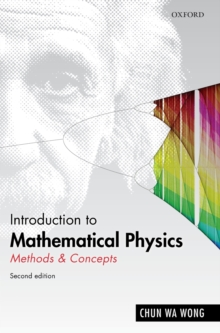Supporting your high street Find out how »
• My Account# Introduction to Mathematical Physics : Methods & Concepts Hardback

#### Description

Mathematical physics provides physical theories with their logical basis and the tools for drawing conclusions from hypotheses.

Introduction to Mathematical Physics explains to the reader why and how mathematics is needed in the description of physical events in space.

For undergraduates in physics, it is a classroom-tested textbook on vector analysis, linear operators, Fourier series and integrals, differential equations, special functions and functions of a complexvariable.

Strongly correlated with core undergraduate courses on classical and quantum mechanics and electromagnetism, it helps the student master these necessary mathematical skills.

It contains advanced topics of interest to graduate students on relativistic square-root spaces and nonlinearsystems.

It contains many tables of mathematical formulas and references to useful materials on the Internet.

It includes short tutorials on basic mathematical topics to help readers refresh their mathematical knowledge.

An appendix on Mathematica encourages the reader to use computer-aided algebra to solve problems in mathematical physics.

A free Instructor's Solutions Manual is available to instructors who order the book for course adoption.

#### Information

• Format: Hardback
• Pages: 736 pages, 102 b/w illustrations
• Publisher: Oxford University Press
• Publication Date:
• Category: Classical mechanics
• ISBN: 9780199641390

£49.99

£43.65

on all orders

###### Pick up orders

from local bookshops